# 怎么用python处理excel文件-Python自动化如何处理excel文件数据

xlrd库 官方地址：https://pypi.org/project/xlrd/

pip install xlrd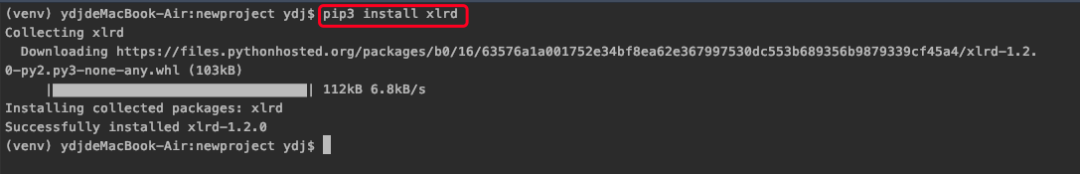Xlrd 库简单的使用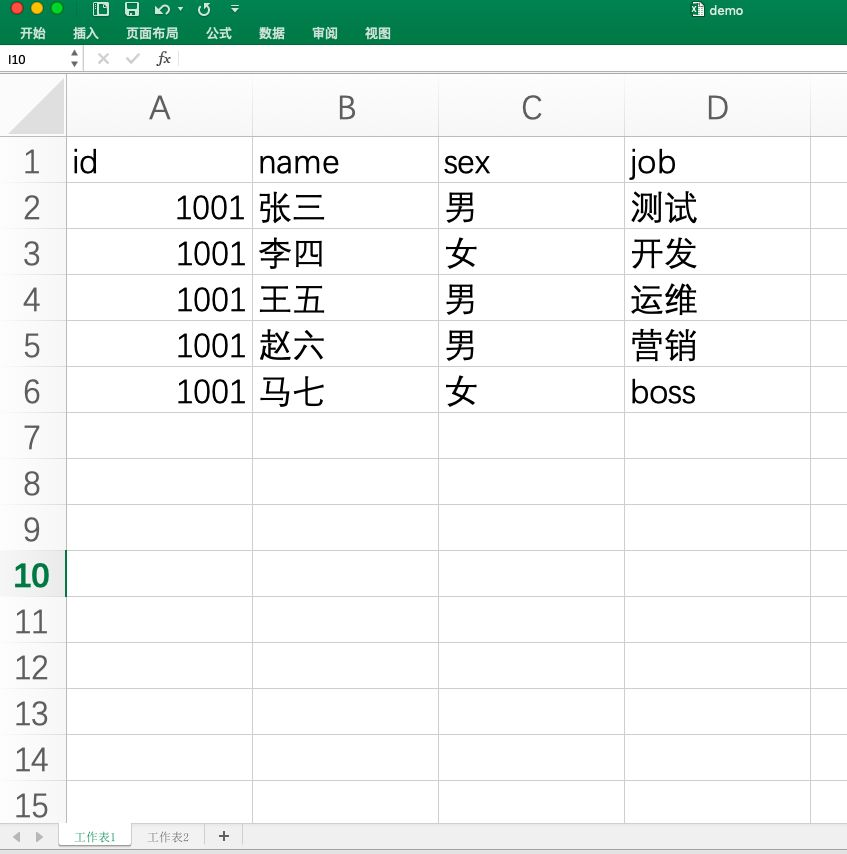# coding=utf-8

importxlrd

# 打开文件

data = xlrd.open_workbook('file/demo.xlsx')

# 查看工作表

data.sheet_names

print("sheets："+str(data.sheet_names))

# 通过文件名获得工作表,获取工作表1

table = data.sheet_by_name('工作表1')

# 打印data.sheet_names可发现，返回的值为一个列表，通过对列表索引操作获得工作表1

# table = data.sheet_by_index(0)

# 获取行数和列数

# 行数：table.nrows

# 列数：table.ncols

print("总行数："+str(table.nrows))

print("总列数："+str(table.ncols))

# 获取整行的值 和整列的值，返回的结果为数组

# 整行值：table.row_values(start,end)

# 整列值：table.col_values(start,end)

# 参数 start 为从第几个开始打印，

# end为打印到那个位置结束，默认为none

print("整行值："+str(table.row_values(0)))

print("整列值："+str(table.col_values(1)))

# 获取某个单元格的值，例如获取B3单元格值

cel_B3 = table.cell(3,2).value

print("第三行第二列的值："+ cel_B3)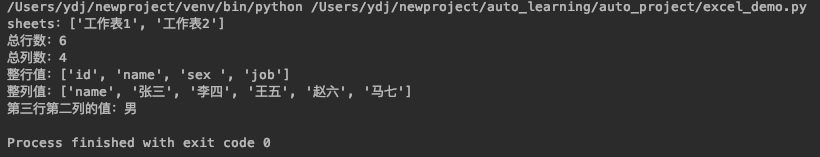# coding=utf-8

importxlrd

data = xlrd.open_workbook(excelFile)

table = data.sheet_by_index(0)

forrowNuminrange(table.nrows):

rowVale = table.row_values(rowNum)

forcolNuminrange(table.ncols):

ifrowNum >0andcolNum ==0:

print(int(rowVale))

else:

print(rowVale[colNum])

print("---------------")

# if判断是将 id 进行格式化

# print("未格式化Id的数据：")

# print(table.cell(1, 0))

# 结果：number:1001.0

if__name__ =='__main__':

excelFile ='file/demo.xlsx'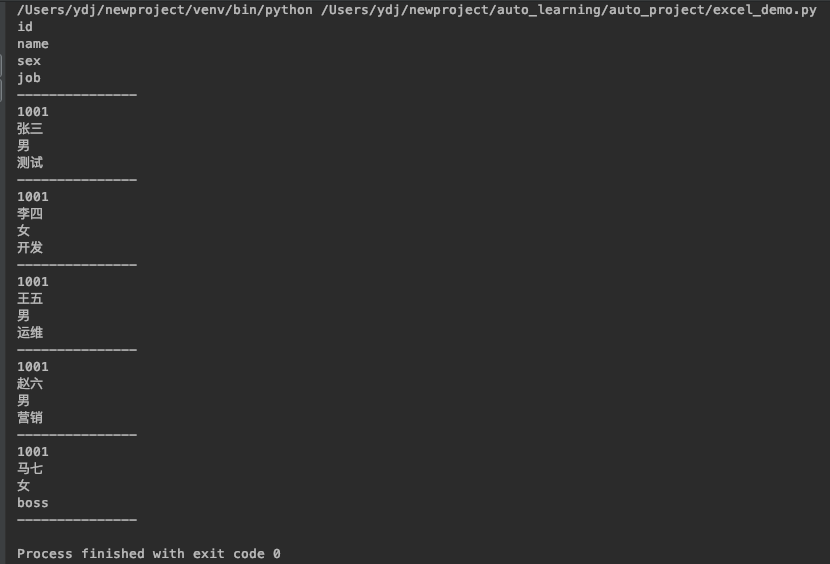# coding=utf-8

importxlrd

data = xlrd.open_workbook(excelFile)

table = data.sheet_by_index(0)

dataFile = []

forrowNuminrange(table.nrows):

# if 去掉表头

ifrowNum >0:

dataFile.append(table.row_values(rowNum))

returndataFile

if__name__ =='__main__':

excelFile ='file/demo.xlsx'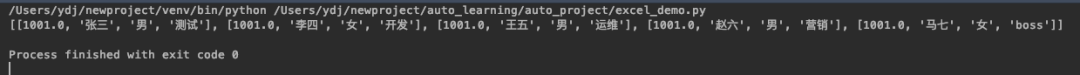RECOMMEND

04-2962908-24
09-16
10-30478
10-3042
10-29350
10-29912